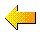ERIC KRAUSE

- © Krause House Info-Research Solutions -ERIC KRAUSE GENEALOGY

ERIC RICHARD KRAUSE
(January 26, 1943, Leamington, Ontario, Canada - )

MY IMMEDIATE ANCESTORS - DETAILS

----------------------------------------

Eric Krause's Pedigree or Ancestry Summary

(ONLY KNOWN MALES WITH KNOWN DESCENDANTS ARE LISTED IN THIS CHART)

 PATERNAL MATERNAL KRAUSE MATHIES Krause b: (1897, 1875, 1845, 1812): m: Kornelsen (b: 1900), Krönert (b: 1875),  Himpel (b: 1849), Koch (b. Unknown) Mathies b: (1893, 1859, 1826, 1797): m: Dück (b: 1897), Baerg (b: 1858), Klassen (b: 1829), Braun (b: 1797), Thiessen (b. After 1760), Loewen (b. c. 1736) Krönert b: (1838; Much Before 1838): m: Gerster (b: 1846), Berthold (b. Unknown) Klassen b: (Much Before 1829): m: Penner (b: Much Before 1829) Gerster b: (1804):  m: Pechel [Peschel] (b: 1806) Braun b: (1770, Unknown): m: Esau (b: 1768) Peschel b: (1774, 1736):  m: Wahl [Wahle] (b: 1783), Dressler (b. 1737) Wahl [Wahle] b: (c. Mid-1700s): m: Braūn (b: Unknown) KORNELSEN DÜCK Kornelsen b: (1876, 1849, 1807, c. 1775, before 1775):  m: Voth (b: 1877), Martens (b: 1851), Walde (b: 1826), Anna [?], [?] Dück b: (1857, 1833, 1802, 1756): m: Ens (b: 1865), Gröning (b: 1823), Loewen (b: 1807), Friesen (b: 1779) Voth b: (c. 1861, c. 1841):  m: Wiens (b: 1854), Unknown (b. Unknown) Ens b: (1824): m: Wiebe (b: 1837) Martens b: (1806, 1784, 1754; 1730):  m: Willms (b: 1811), Enns (b: 1774), Janzen (b: c. 1758), Unknown (b: before 1736) Gröning b: (c.1796, 1771): m: Balzer (b: 1800), Friesen (b: c. 1780) Walde b: (died 1876):  m: Bourne (b: 1802) Wiebe b: (1796, 1746); m: Unknown (b: 1803), Dyck (b: 1774 - Perhaps 1766) Bourne b: (before 1775): m. Doerksen (b: 1773) Wiens b: (1819, 1781, 1760):  m: Janzen (b: 1827), Reimer (b: 1782), Klassen (b: 1754) Janzen b: (1792, 1770, c. 1735 ):  m: Hamm (b: 1793), Unknown (b: c. 1774), Unknown (b: c. 1736) Hamm b: (c. 1759): m: Goossen [Goosen] (c. 1761) Willms b: (1784; 1759; 1730):  m: Wiens (b: 1789), Klassen (Klaasen) (b: 1752), Thun (Thunen) (b: 1731) Enns b: (1742):  m: Unknown (b: 1739) Thun b: Thunen) (before 1711): m: Unknown (b: Much Before 1731) Janzen b: (1729): m: Unknown (b: before 1737)

----------------------------------------

ARE YOU A COUSIN OR COUSIN REMOVED ?

IF YOU CAN TRACE YOUR ANCESTOR BACK TO ONE OF THE FOLLOWING
PAIRS OF GRAND PARENTS LISTED BELOW, YES YOU ARE: